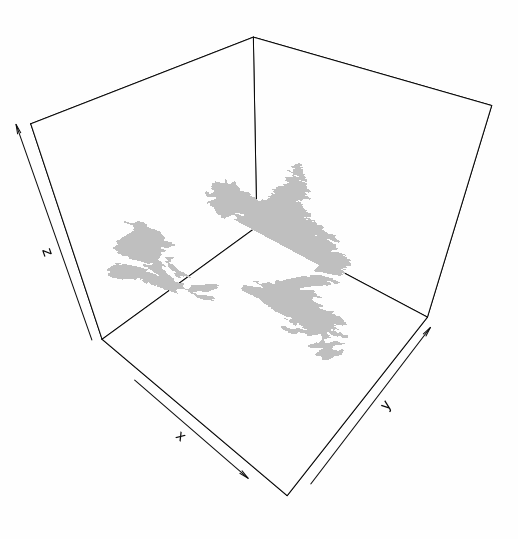# 3D Visualization of Shapefiles in R

## With a lot of help from Ananda Mahto

Posted by Bhargav Rao on February 11, 2016

3D Visualization of ESRI Shapefiles in R has always been a difficult process. Not many have tried to visualize geospatial data in 3D. I have utilized `maptools` and `plot3D` for the following visualization. So we include the libraries

``````library(maptools)
library(plot3D)
``````

First we use the `readShapeSpatial` function from `maptools` to read the shapefile. Remeber to point it to the `.shp` file.

``````ShapeFile <- readShapeSpatial('Test.shp')
``````

I have used a shapefile of Atlantic Canada in my example below, you can use the same from here

The `S4` object returned from `readShapeSpatial` contains a slot called `polygon`. This helps us get the co-ordinates to plot the different polygons.

``````Polygons <- slot(ShapeFile,"polygons")
``````

Using `lapply`, we can extract the coordinate values and the Polygon ID from the slot. This is done as follows.

``````temp <- do.call(rbind, lapply(Polygons, function(x) slot(slot(x, "Polygons")[], "coords")))
``````

Now we create a dummy plot

``````polygon3D(temp[, 1], temp[, 2], rep(0, nrow(temp)), col="transparent")
``````

Finally we use `polygon3D` to draw the polygons.

``````for (i in seq_along(Polygons)) {
temp <- slot(slot(Polygons[[i]], "Polygons")[], "coords")
polygon3D(temp[, 1], temp[, 2], rep(0, nrow(temp)), add = TRUE)
}
``````

We now have our nice map.You can download the complete R code by clicking here